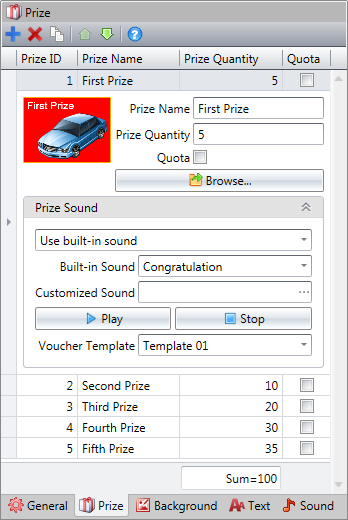﻿ 2.2.1 Prize Quantity and Quota

# 2.2.1 Prize Quantity and Quota

Top  Previous  Next
 If "Quota" is checked, the maximum quantity of prizes can be drawn equals the quantity set to this prize. For example, as shown in the following image, prize quantity of "First Prize" is 5, sum of all prize quantity is 100, ➢If "Quota" is unchecked, the prize "First Prize" will be drawn 5 times if press Space key 100 times, the prize "First Prize" will be drawn 10 times if press Space key 200 times.➢If "Quota" is checked, the prize "First Prize" will be drawn 5 times if press Space key 100 times, the prize "First Prize" will still be drawn 5 times if press Space key 200 times.